Java帮帮-微信公众号Java帮帮-微信公众号将分享做到极致 支付宝小程序支付宝小程序支付也能阅读 程序员生活志-公众号程序员生活志-公众号程序员生活学习圈，互联网八卦黑料

 编程资讯
 Java
 程序员段子
 程序人生
 秒杀专栏
 名企资讯
 运维监控
 人事面试
 技术面试
 大禹编程
 UI
 SpringBoot
 SpringCloud(F版)
 SpringCloud(D版)
 spring
 Docker
 Dubbo
 IntelliJ IDEA
 Linux
 Nginx
 Tomcat
 Quartz
 微信小程序
 阿里云
 Python
 Java案例
 小程序源码
 Java源码
 手机资讯
 Sql Server
 MySQL
 MongoDB
 前端开发
 node.js
 CSS
 移动端
 jQuery
 Sublime Test
 JavaScript
 HTML
 Vue.js
 HTTPS
 分布式事物
 Redis
 JSON
 DevOps
 情感人生
 黑科技
 Oracle
 Git
 微服务
 大数据
 职业生涯
 人工智能
 Keras教程
 RocketMQ
 PyTorch教程
 Spring Security

# 第十六天 常用API-Date&DateFormat&Calender&System&Math&基本类型包装类&正则【悟空教程】

58# 第1章 常用API

## 1.1 Date类

### 1.1.1 概念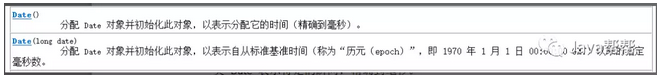//创建日期对象，把当前的毫秒值转成日期对象

Date date = new Date(1607616000000L);

System.out.println(date);

//打印结果：Fri Dec 11 00:00:00 CST 2020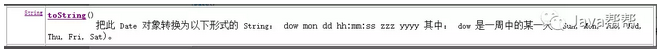### 1.1.2 常见方法

public Date() //返回当前时间

public Date(long date) //返回指定毫秒值的日期对象

public long getTime()   //获取当前时间对象的毫秒值

public void setTime(long time)  //设置时间毫秒值

import java.util.Date;

/*

* Date是日期类  表示一个瞬间

* 构造方法：

* public Date() //返回当前时间

* public Date(long date) //返回指定毫秒值的日期对象

* 普通方法:

* public long getTime()   //获取当前时间对象的毫秒值

* public void setTime(long time)  //设置时间毫秒值

*/

public class Demo01_Date {

public static void main(String[] args) {

Date date = new Date();

System.out.println(date);

//获取当前时间对象的毫秒值

long time = date.getTime();

System.out.println(time);

//设置date的毫秒值为0

date.setTime(0);

System.out.println(date);

}

}

### 1.1.3 注意事项

Date是早期版本出现的，其API中有大量的过时方法，其许多方法由更为新的类Calendar类再次实现。

Date的默认格式为西方的月日年的，而非中国的年月日。

## 1.2 DateFormat类

### 1.2.1 概念

DateFormat是用于日期格式化类，即将一个Date对象转换为一个符合指定格式的字符串，也可以将一个符合指定格式的字符串转为一个Date对象。

DateFormat是抽象类，我们需要使用其子类SimpleDateFormat。

### 1.2.2 常见方法

public  SimpleDateFormat()   //使用默认格式创建格式化对象

public  SimpleDateFormat(String pattern)   //使用指定格式创建格式化对象

public  final  String  format(Date date)  //将Date转为字符串

public  Date  parse(String source)   //将字符串转为Date（转换时，该String要符合指定格式，否则不能转换）

import java.text.DateFormat;

import java.text.ParseException;

import java.text.SimpleDateFormat;

import java.util.Date;

/*

*DateFormat

*DateFormat是抽象类，我们需要使用其子类SimpleDateFormat

*

*xxxxxxxx日  xx:xx:xx

*

*日期 --指定格式字符串

*public  final  String  format(Date date) //Date转为字符串

*

*指定格式字符串--日期

*public Date parse(String source) throws ParseException  //将字符串转为Date

*

*/

public class Demo02_DateFormat {

public static void main(String[] args) throws ParseException {

//创建当前时间日期对象

Date now = new Date();

//创建日期格式化DateFormat对象

//使用默认格式创建格式化对象

// DateFormat df = new SimpleDateFormat();

//使用指定格式创建格式化对象

DateFormat df = new SimpleDateFormat("yyyyMMdd日  HH:mm:ss");

//调用format方法将日期转换为字符串

String sDate = df.format(now);

System.out.println(now);

System.out.println(sDate);

System.out.println("--------------------------------------------");

//1949-10-01 求给定字符串日期的毫秒值

String birth = "1949-10-01";

//创建日期格式化对象

DateFormat df2 = new SimpleDateFormat("yyyy-MM-dd");

//调用parse方法将字符串转换为日期对象

Date d = df2.parse(birth);

//调用Date类的getTime方法获取毫秒值

System.out.println(d.getTime());

}

}

## 1.3 Calendar类

### 1.3.1 概念

Calendar是日历类，在Date后出现，替换掉了许多Date的方法。该类将所有可能用到的时间信息封装为字段值，方便获取。

Calendar为抽象类，由于语言敏感性，Calendar类在创建对象时并非直接创建，而是通过静态方法创建，将语言敏感内容处理好，再返回子类对象，如下：

Calendar类静态方法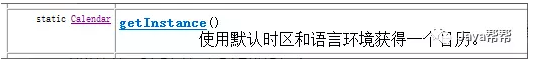Calendar c = Calendar.getInstance();  //返回当前日历对象

public static Calendar {

Calendar c = new GregorianCalendar();

return c;

}

### 1.3.2 常见方法

public static Calendar getInstance()    //获取日期对象

public int get(int field)       //获取时间字段值，字段参见帮助文档

• YEAR 年

• MONTH 月，从0开始算起，最大11；0代表1月，11代表12月。

• DATE 天

• HOUR 时

• MINUTE分

• SECOND秒

public void add(int field,int amount)    //指定字段增加某值

public final void set(int year,int month,int date)  //设置年月日，可查看对应重载

public final Date getTime()      //获取该日历对象转成的日期对象

import java.util.Calendar;

/*

* Calendar 日历类 也表示一个瞬间

*

* public static Calendar getInstance() //获取日期对象

* Calendar rightNow = Calendar.getInstance();  //返回当前时间

*

* public int get(int field)     //获取时间字段值，字段参见帮助文档

* public void add(int field,int amount)   //指定字段增加某值

*

* public final void set(int year,int month,int date) //设置年月日，可查看对应重载

*

* public final Date getTime()     //获取该日历对象转成的日期对象

*/

public class Demo01_Calendar {

public static void main(String[] args) {

//获取日期对象

Calendar rightNow  = Calendar.getInstance();

System.out.println(rightNow);

//获取时间字段值，字段参见帮助文档

int i = rightNow.get(Calendar.YEAR);

System.out.println(i);

System.out.println(rightNow.get(Calendar.MONTH));

System.out.println(rightNow.get(Calendar.DATE));

System.out.println(rightNow.get(Calendar.DAY_OF_MONTH));

//给指定年份 减1

int nian = rightNow.get(Calendar.YEAR);

System.out.println(nian);

rightNow.set(2017, 9, 10);

System.out.println(rightNow.get(Calendar.YEAR));

System.out.println(rightNow.get(Calendar.MONTH));

System.out.println(rightNow.get(Calendar.DATE));

//日历对象获取毫秒值

long time = rightNow.getTime().getTime();

long timeInMillis = rightNow.getTimeInMillis();

System.out.println(time);

System.out.println(timeInMillis);

}

}

## 1.4 练习

### 1.4.1 获取指定年份（int）的2月份有多少天

import java.util.Calendar;

/*

* 获取指定年份（int）的2月份有多少天

*/

public class Test01 {

public static void main(String[] args) {

int year = 2016;

int day = showDay(year);

System.out.println(day);

}

/*

* 获取到指定年份的31

* 1 -1 获取到2月 份最后1

*

*

*/

public static int showDay(int year) {

//创建日历对象

Calendar c = Calendar.getInstance();

//获取到指定年份的31

c.set(year, 2, 1);

//1 -1 获取到2月 份最后1

//获取到当月天数

int day = c.get(Calendar.DAY_OF_MONTH);

return day;

}

}

### 1.4.2 给定一个字符串 生日 格式 1949-10-01  计算 你出生了多少天

import java.text.DateFormat;

import java.text.ParseException;

import java.text.SimpleDateFormat;

import java.util.Date;

/*

* 给定一个字符串 生日 格式 1949-10-01  计算 你出生了多少天

*/

public class Test02 {

public static void main(String[] args) throws ParseException {

String birth = "2016-07-01";

int day = showDay(birth);

System.out.println(day);

}

/*

* 将字符串转换成日期   获取毫秒值1

* 创建DateFormat对象  调用parse方法将字符串转换成日期

*

*

* 创建当前时间对象  获取毫秒值2

*

* （毫秒值2 - 毫秒值1 /1000/60/60/24

*

*/

public static int showDay(String birth) throws ParseException {

//创建DateFormat对象

DateFormat df = new SimpleDateFormat("yyyy-MM-dd");

//调用parse方法将字符串转换成日期

Date parse = df.parse(birth);

//获取给定时间的毫秒值

long time1 = parse.getTime();

//创建当前时间对象  获取毫秒值2

long time2 = new Date().getTime();

//（毫秒值2 - 毫秒值1 /1000/60/60/24

long day = (time2-time1)/1000/60/60/24;

return (int)day;

}

}

## 1.5 System类

### 1.5.2 常用方法

public static void gc()    //用来运行JVM中的垃圾回收器，完成内存中垃圾的清除

public static void exit(int status)   //用来结束正在运行的Java程序。参数传入一个数字即可。通常传入0记为正常状态，其他为异常状态

public static long currentTimeMillis()  //获取当前系统时间与1970年01月01日00:00点之间的毫秒差值

public static Properties getProperties()  //用来获取指定键(字符串名称)中所记录的系统属性信息

import java.util.Calendar;

import java.util.Date;

import java.util.Properties;

/*

* public static void gc()   //回收垃圾

* public static void exit(int status) //退出程序，0为正常状态，其他为异常状态

* public static long currentTimeMillis() //获取当前时间毫秒值

* public static Properties getProperties() //获取某个属性信息

*/

public class Demo_System {

public static void main(String[] args) {

//构造方法被私有 不能创建对象

//System sy = new System();

//public static void exit(int status) //退出程序，0为正常状态，其他为异常状态

// System.out.println("我要退出了！");

// System.exit(0);

// System.out.println("我已经退出了！");

//public static long currentTimeMillis() //获取当前时间毫秒值

long timeMillis = System.currentTimeMillis();

long time = new Date().getTime();

long timeInMillis = Calendar.getInstance().getTimeInMillis();

System.out.println(timeMillis);

for (int i = 0; i < 1000; i++) {

System.out.println("i love java");

}

long timeMillis2 = System.currentTimeMillis();

System.out.println(timeMillis2-timeMillis);

//public static Properties getProperties() //获取某个属性信息

Properties properties = System.getProperties();

System.out.println(properties);

}

}

### 1.5.3 注意事项

System类不能手动创建对象，因为构造方法被private修饰，阻止外界创建对象。System类中的都是static方法，类名访问即可。在JDK中，有许多这样的类。

## 1.6 Math类

### 1.6.1 概念

Math 类是包含用于执行基本数学运算的方法的数学工具类，如初等指数、对数、平方根和三角函数。

### 1.6.2 常用方法

public static int abs(int a)     //绝对值

public static double ceil(double a)    //向上取整

public static double floor(double a)   //向下取整

public static int max/min(int a,int b)    //取最大最小值

public static double pow(double a,double b)  //a^b

public static double random()     //产生随机数

public static int round(float a)    //四舍五入

/*

* 数学工具类  不能创建对象 所有方法全部是static修饰  类名直接调用

*

* public static int abs(int a)   //绝对值

* public static double ceil(double a)   //向上取整

* public static double floor(double a) //向下取整

* public static int max/min(int a,int b) //取最大最小值

* public static double pow(double a,double b) //a^b

* public static double random()   //产生随机数

* public static int round(float a)   //四舍五入

*/

public class Demo01_Math {

public static void main(String[] args) {

System.out.println(Math.abs(-10));//绝对值

System.out.println("-----------------------------");

//四舍五入

System.out.println(Math.round(10.1));//10

System.out.println(Math.round(10.8));//11

System.out.println(Math.round(-10.1));//-10

System.out.println(Math.round(-10.8));//-11

System.out.println("---------------------------");

//向上取整

System.out.println(Math.ceil(10.1));//11

System.out.println(Math.ceil(10.8));//11

System.out.println(Math.ceil(-10.1));//-10

System.out.println(Math.ceil(-10.8));//-10

System.out.println("-------------------------------");

//向下取整

System.out.println(Math.floor(10.1));//10

System.out.println(Math.floor(10.8));//10

System.out.println(Math.floor(-10.1));//-11

System.out.println(Math.floor(-10.8));//-11

System.out.println("----------------------------------");

//public static int max/min(int a,int b) //取最大最小值

System.out.println(Math.max(10.1, 20.2));//20.2

System.out.println(Math.min(10.1, 20.2));//10.1

System.out.println("-------------------------------------");

//public static double pow(double a,double b) //ab次方

System.out.println(Math.pow(2, 8));

System.out.println("-----------------------------------");

//public static double random()   //产生随机数

System.out.println(Math.random());// 0.1之间随机小数 包含0 不包含1

System.out.println("-------------------------------------");

}

}

## 1.7 基本类型包装类

### 1.7.1 概念

Java中提供了相应的对象来解决该问题，基本数据类型对象包装类：java将基本数据类型值封装成了对象。封装成对象有什么好处？可以提供更多的操作基本数值的功能。

8种基本类型对应的包装类如下：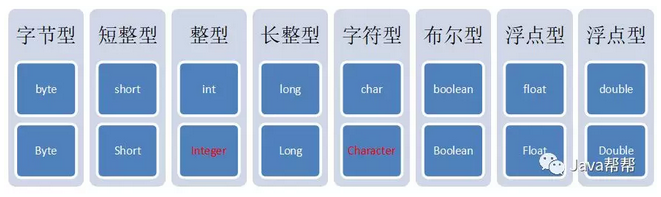### 1.7.2 常用方法

• 字符串→基本类型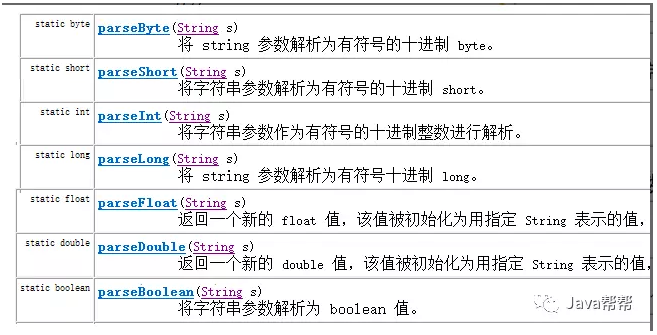parseXXX(String s);其中XXX表示基本类型，参数为可以转成基本类型的字符串，如果字符串无法转成基本类型，将会发生数字转换的问题 NumberFormatException

System.out.println(Integer.parseInt("123") + 2);

//打印结果为 125

• 基本类型→字符串

int i = 10;

String s = i+””;

### 1.7.3 自动装箱拆箱

• 自动拆箱：对象转成基本数值

• 自动装箱：基本数值转成对象

Integer i = 4;//自动装箱。相当于Integer i = Integer.valueOf(4);

i = i + 5;//等号右边：将i对象转成基本数值(自动拆箱) i.intValue() + 5; 加法运算完成后，再次装箱，把基本数值转成对象。

Integer a = 10;    //10这个基本类型值自动装箱成了引用类型

int b = new Integer(“10”);  //引用数据类型自动拆箱成了基本类型

Integer c = b + a; //计算时，a自动拆箱为基本类型，结果自动装箱成引用类型。

import java.util.ArrayList;

/*

* 基本类型包装类

* int == Integer

*

* 字符串→基本类型

* 任何一种基本类型包装类都有一个parseXxx(String data)方法。如IntegerParseInt方法。

*

* public static int parseInt(String s) throws NumberFormatException

*

* 基本类型--字符串

* 基本类型+""

*/

public class Demo01_Integer {

public static void main(String[] args) {

String s = "123";

int i = Integer.parseInt(s);

System.out.println(i);

//-------------------------------------

String ss = i+"";

System.out.println(ss);

System.out.println("-------------------------");

int a = 10;

int b = 20;

System.out.println(a-b);

Integer in1 = new Integer(10);

Integer in2 = new Integer(20);

//自动拆箱

System.out.println(in1- in2);

ArrayList<Integer> list = new ArrayList<Integer>();

//自动装箱

// Integer integer = list.get(0);

//自动拆箱

int i2 = list.get(0);

System.out.println("------------------------");

Integer aa = 10;    //10这个基本类型值自动装箱成了引用类型

int bb = new Integer("10");  //引用数据类型自动拆箱成了基本类型

Integer c = bb + aa; //计算时，a自动拆箱为基本类型，结果自动装箱成引用类型。

}

}

## 1.8 简单正则表达式

### 1.8.1 概念

String类中，使用正则表达式匹配完整字符串的方法为：

public boolean matches(String regex)

regex字符串为匹配规则

String  data = “aaa@163.com”;

boolean  result = data.matches(“\\w+@\\w{2,7}\\.\\w{2,3}”);  //返回结果为true

String  data2 = “itheima.cn”;

boolean  result2 = data2.matches(“\\w+@\\w{2,7}\\.\\w{2,3}”);  //返回结果为false

import java.util.Scanner;

/*

* 简单正则表达式

*

* 正则表达式：匹配指定规则的字符串

*

* 判断一个字符串

* 1.长度大于等于5小于等于15

* 2.数字开头 不包含0

*

*/

public class Demo01 {

public static void main(String[] args) {

Scanner sc = new Scanner(System.in);

String s = sc.nextLine();

boolean flag = check(s);

boolean flag2 = check2(s);

System.out.println(flag);

System.out.println(flag2);

}

public static boolean check2(String s){

// String regex="[1-9].{4,14}";

// boolean b = s.matches(regex);

// return b ;

return s.matches("[1-9].{4,14}");

}

public static boolean check(String s) {

boolean flag = true;

//判断长度

if(s.length()>=5 &&s.length()<=15){

char c = s.charAt(0);

//判断数字开头 不包含0

if(!(c>='1'  && c<='9')){

flag = false;

}

}else{

flag = false;

}

return flag;

}

}

### 1.8.2 字符串中涉及正则的常用方法

public boolean matches(String regex)     //完整字符串匹配规则(判断字符串是否匹配给定的规则)

/*

* public boolean matches(String regex)    判断调用方法的字符串与regex字符串是否匹配

*

* 字符类

* [abc] ab c（简单类）

* [^abc] 任何字符，除了 ab c（否定）

* [a-zA-Z] a z A Z，两头的字母包括在内（范围）

* 预定义字符类

* . 任何字符（与行结束符可能匹配也可能不匹配）

* \w 单词字符：[a-zA-Z_0-9]

* 26个字母大小写  数字 _

*

* \b 单词边界

* abc?bcda aaa

* Greedy 数量词

* X? X，一次或一次也没有

* X* X，零次或多次

* X+ X，一次或多次

* X{n} X，恰好 n

* X{n,} X，至少 n

* X{n,m} X，至少 n 次，但是不超过 m

*

*/

public class Demo02 {

public static void main(String[] args) {

String s ="aaaac";

String regex = "a{1,3}c";

boolean matches = s.matches(regex);

System.out.println(matches);

}

}

1: 要求必须是5-15位数字

2: 0不能开头

String qq = "604154942";

String regex = "[1-9][0-9]{4,14}";

boolean flag2 = qq.matches(regex);

1：要求为11位数字

2：第1位为1，第2位为34578中的一个，后面9位为09之间的任意数字。

String phone = "18800022116";

String regex = "1[0-9]{9}";

boolean flag = phone.matches(regex);

public String[] split(String regex)      //将符合规则的字符串作为切割符切割

public String replaceAll(String regex,String replacement) //将符合规则的字符串替换为新字符串

/*

* public String[] split(String regex) //将符合规则的字符串作为切割符切割

* public String replaceAll(String regex,String replacement) //将符合规则的字符串替换为新字符串

*/

public class Demo03 {

public static void main(String[] args) {

String girlName = "姗姗.慧慧.柳柳.莲莲.糖糖 ";

String[] girlNames = girlName.split("\\.");

// System.out.println(girlNames);

// System.out.println(girlNames);

// System.out.println(girlNames);

// System.out.println(girlNames);

// System.out.println(girlNames);

for (int i = 0; i < girlNames.length; i++) {

String string = girlNames[i];

System.out.println(string);

}

String replaceAll = girlName.replaceAll("", "岩岩");

System.out.println(replaceAll);

}

}

String s = "Hello12345World6789012";

String regex = "[0-9]";

String result = s.replaceAll(regex, "*");

### 1.8.3 补充内容:正则表达式的匹配规则

String regex = ".*\\b[abc]\\b.*";

String str = "abc a bbc";

boolean flag = str.matches(regex);

# 第2章 本日自习作业：

## 2.1 知识点相关题

### 2.1.5 包装类

1) 掌握8种基本数据类型的包装类；

2) 掌握String和这8种类型的转换；

1) 写出8种基本数据类型对应的“包装类”；

2) 定义类Test1，定义main()方法，按以下要求编写代码：

• 定义字符串s1，初始值：”100”；

• 将s1转换为int值

• 将s1赋值为”3.14”；

• 将s1转换为double值

• 将s1赋值为”你”；

• 将s1转换为char值

• 将s1赋值为：”true”

• 将s1转换为boolean值

• 定义int变量v1，初始值：200

• 将v1转换为String

• 定义double变量v2，初始值：3.14

• 将v2转换为String

### 2.1.6 System类

1) 掌握System类的常用方法；

1) 定义类Test2，定义main()方法，按以下要求编写代码：

• 定义long类型变量start，存储当前系统时间毫秒值；

• 使用for循环打印9999内的所有值；

• 定义long类型变量end，存储当前系统时间毫秒值；

• 打印循环执行时间：end – start

• 调用exit()结束程序；

2) 定义类Test3，定义main()方法，按以下要求编写代码：

• 获取并打印你电脑上Java的安装目录；

• 获取并打印你电脑上Java运行时的版本号；

• 获取并打印你电脑上操作系统的名称和版本号；

### 2.1.7 Math类

1) 掌握Math类的常用方法；

1) 将讲义上所有Math方法的代码打一遍；写上注释。关注每种方法的作用；

## 2.2 代码题

### 2.2.1 分别用几种不同的格式输出当前日期.格式参考如下:

 public class Test {public static void main(String[] args) {//1、获取当前日期对象Date d = new Date();//2、分别定义3种日期格式化对象，用于表示3种日期格式SimpleDateFormat sdf1 = new SimpleDateFormat("yyyy/MM/dd HH:mm:ss");SimpleDateFormat sdf2 = new SimpleDateFormat("yyyy年MM月dd日  今天是今年的第D天");SimpleDateFormat sdf3 = new SimpleDateFormat("yyyy.MM.dd E");//3、分别使用3个格式化对象格式化日期String f1 = sdf1.format(d);String f2 = sdf2.format(d);String f3 = sdf3.format(d);//4、分别打印3个格式化出来的结果System.out.println(f1);System.out.println(f2);System.out.println(f3);}}

### 2.2.2 键盘输入指定格式(yyyy-MM-dd)的任意日期,显示出对应的是星期几.

 public class Test {public static void main(String[] args) throws ParseException {//1、让用户按格式输入日期System.out.println("亲，请输入日期：（格式为：yyyy-MM-dd）");String s = new Scanner(System.in).next();//2、定义日期格式化对象，用于将用户输入的字符串转换为日期对象SimpleDateFormat sdf1 = new SimpleDateFormat("yyyy-MM-dd");//3、将字符串转换为日期对象Date date = sdf1.parse(s);//4、定义日期格式化对象，用于将日期对象转换为指定的字符串形式的对象SimpleDateFormat sdf2 = new SimpleDateFormat("E");//5、解析日期对象为字符串形式String f2 = sdf2.format(date);//6、打印格式化出来的结果System.out.println(f2);}}

### 2.2.3 计算字符串中所有数字的平均值.题目考察字符串的解析和引用类型转换.

String s= " 第一组得分：92.8，第二组得分：88.8，第三组得分：95.4";

 public class Test {public static void main(String[] args) throws ParseException {String s= "第一组得分:92.8,第二组得分:88.8,第三组得分:95.4";//1、定义正则表达式，匹配数字和逗号以外的字符，并使用replaceAll方法，将数字以外的字符替换掉；String re = "第[一二三]组得分:";String s1 = s.replaceAll(re, "");//s1=92.8,88.8,95.4//2、将s1字符串使用，切分，即可得到一个字符串数组，数组的每个元素就是我们需要的数字String[] split = s1.split(",");//3、迭代数组，并将数组中的每一个元素都转换为double类型后，求和double sum =0;for(String sp : split){double d = Double.parseDouble(sp);sum+=d;}//4、求平均数double pingJun = sum/split.length;System.out.println("字符串："+s+"中的数字的平均数为："+pingJun);}}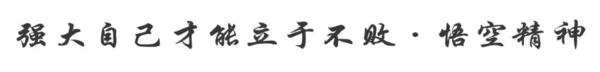Java帮帮学习群生态

Java帮帮学习群生态

 Java学习群Java学习群与大牛一起交流 大数据学习群大数据学习群在数据中成长 九点编程学习群九点编程学习群深夜九点学编程 python学习群python学习群人工智能,爬虫
Java帮帮生态承诺

Java帮帮生态承诺